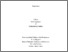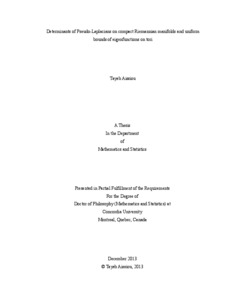Title:

# Determinants of Pseudo-Laplacians on compact Riemannian manifolds and uniform bounds of eigenfunctions on tori

Aissiou, Tayeb (2013) Determinants of Pseudo-Laplacians on compact Riemannian manifolds and uniform bounds of eigenfunctions on tori. PhD thesis, Concordia University.Preview
Text (application/pdf)
Aissiou_PhD_S2014.pdf - Accepted Version
562kB

## Abstract

In the first part of this thesis, we derive comparison formulas relating the zeta-regularized determinant of an arbitrary self-adjoint extension of the Laplace operator with domain consisting of smooth functions compactly supported on the complement of a point $P$, to the zeta-regularized determinant of the Laplace operator on $X$. Here $X$ is a compact Riemannian manifold of dimension 2 or 3; $P\in X$. In the second part, we provide a proof of a conjecture by Jakobson, Nadirashvili, and Toth stating that on an n-dimensional flat torus, the Fourier transform of squares of the eigenfunctions $|phi_j|^2$ of the Laplacian have uniform $l^n$ bounds that do not depend on the eigenvalue $\lambda_j$. The thesis is based on two published papers that can be found in the bibliography.

Divisions: Concordia University > Faculty of Arts and Science > Mathematics and Statistics Thesis (PhD) Aissiou, Tayeb Concordia University Ph. D. Mathematics 2013 Kokotov, Alexey and Korotkin, Dmitri Determinants, Pseudo-Laplacian, Laplacian, Eigenfunctions, Eigenvalues, uniform bounds, L^p, compact manifolds, determinants of Laplacian, self-adjoint, extensions, zeta function, regularized determinant, geometric lemma, 978211 TAYEB AISSIOU 16 Jun 2014 14:06 18 Jan 2018 17:46
All items in Spectrum are protected by copyright, with all rights reserved. The use of items is governed by Spectrum's terms of access.

Repository Staff Only: item control pageResearch related to the current document (at the CORE website)
Back to top